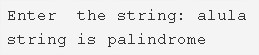# C Program To Check A String Is Palindrome Or Not | C Programs

C program to check whether a string is a palindrome or not – In this article, we will detail in on the multiple ways to check whether a string is a palindrome or not in C programming.

Suitable examples and sample programs have also been added so that you can understand the whole thing very clearly. The compiler has also been added with which you can execute it yourself.

Also check list of over 500+ C Programs with source codes.

The ways used in this piece are as follows:

• Using Standard Method
• Using Function
• Using Recursion
• Using String Library Function

A string is nothing but an array of characters. The value of a string is determined by the terminating character. Its value is considered to be 0.As you can see in the image uploaded above, you need to enter the concerned string first.

The string entered here is ‘alula‘.

As it is clearly visible, ‘alula’ is a palindrome.

Thus, to check the same in C programming is as follows:

## Using Standard Method

1. If the original string is equal to reverse of that string, then the string is said to be a palindrome.

2)Read the entered string using gets(s).

3) Calculate the string length using string library function strlen(s) and store the length into the variable n.

4) i=0,c=0.

Compare the element at s[i] with the element at s[n-i-1].If both are equal then increase the c value.

Compare the remaining characters by increasing i value up to i<n/2.

5) If the number of characters compared is equal to the number of characters matched then the given string is the palindrome.

Output:

### Using Function

1. The main() function calls the checkpalindrome(char *s) by passing the string as an argument.

2) c=0,calculate the string length n using strlen(s) library function.

3) For loop iterates from i=0 to i<length of the string/2,

If the element at s[i] is equal to element at s[n-i-1] then increase the c value.

4) If the number of elements compared is equal to the number of elements matched then the function return 1, otherwise it returns 0.

Output:

#### Using Recursion

1. The main() calls the function checkpalindrome(char *s).

2) The function checkpalindrome(char * s)i=0,c=0.Calculate the string length n using strlen(s).

a) i=0,c=0.Calculate the string length n using strlen(s).

b) if i<length of the string/2

If the element at s[i] is equal to the element at s[n-i-1] then increase the c value and i value.

The function calls itself.

The function calls itself recursively until i<n/2.

c) If i!<length of the string/2,

If i=c then this function returns 1 otherwise it returns 0.

3) If the returned value is 1 then print the given string is a palindrome. If the returned value is 0 then print the string is not a palindrome.

Output:

##### Using String Library Function
1. Read the string s using gets(s1) and store into the variable s1.

Using library functions we are comparing the two strings.The string library functions are available at string.h header file.

2) Copy the string s1 to s2 using the library function strcpy(s2,s1).

3) Reverse the string s2 using the library function strsev(s2).

4) Then compare the two strings s1 and s2 using the library function strcmp(s1,s2).

5) If the function strcmp(s1,s2) returns 0 then  print the string is palindrome.Otherwise, print the string is not a palindrome.

Output:
x

## C Program To Print Number Of Days In A Month | Java Tutoring

C program to input the month number and print the number of days in that ...